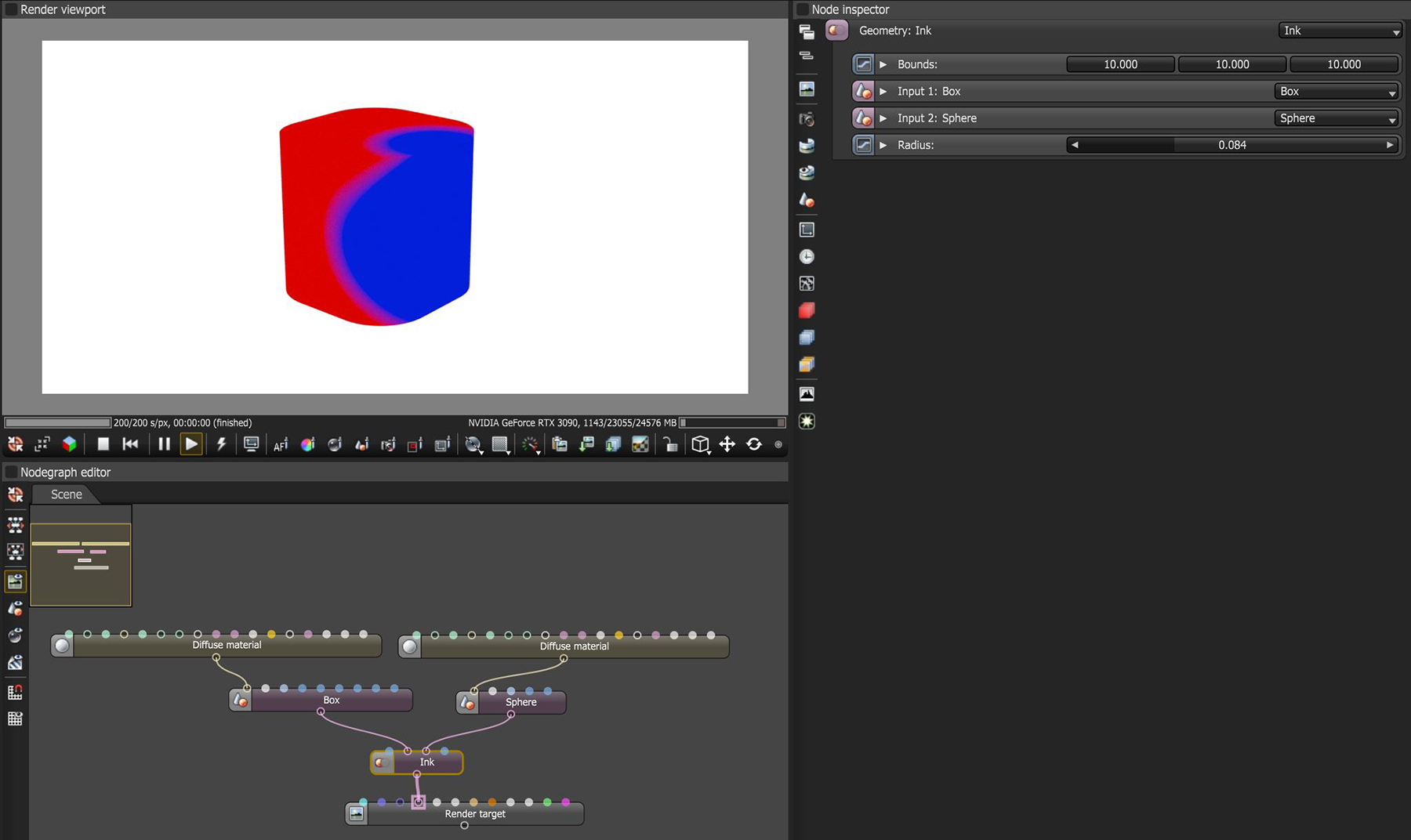# Ink

The Ink Vectron operator will place the second input's material on the first input where the two Vectron objects intersect (figure 1). The Radius parameter will blur the transition between the two materials.Figure 1: The second input's material on the Ink node is being placed on the first input's surface where the two signed distance fields overlap